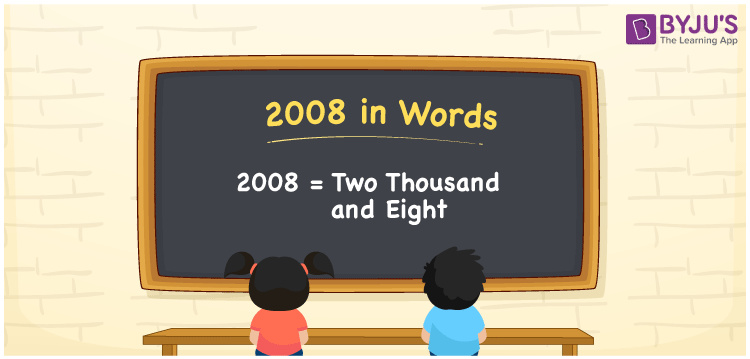# 2008 in Words

We can write 2008 in words as Two thousand and eight. Suppose you purchased a portable mini-fan that costs Rs. 2008, then you can say, “I purchased a portable mini-fan worth Two thousand and eight rupees”. Here, the word form “Two thousand and eight” represents the name for the number 2008. Let’s understand how to convert the cardinal number 2008 into words here, in this article.

 2008 in words Two thousand and eight Two thousand and eight in Numbers 2008

## 2008 in English Words

We generally express numbers in words using the English alphabet. Thus, we can write 2008 in English as “Two thousand and eight”.## How to Write 2008 in Words?

The below table depicts the place value chart for the number 2008. This chart shows the place value for all the four digits of the number 2008.

 Thousands Hundreds Tens Ones 2 0 0 8

Here, ones = 8, tens = 0, hundreds = 0, thousands = 2

Thus, by expanding these digits, we get;

2 × Thousand + 0 × Hundred + 0 × Ten + 8 × One

= 2 × 1000 + 0 × 100 + 0 × 10 + 8 × 1

= 2000 + 8

= Two thousand + Eight

= Two thousand and eight

Therefore, 2008 in words = Two thousand and eight.

As we know, 2008 is a natural number that precedes 2009 and succeeds 2007.

2008 in words – Two thousand and eight

Is 2008 an odd number? – No

Is 2008 an even number? – Yes

Is 2008 a prime number? – No

Is 2008 a composite number? – Yes

Is 2008 a perfect square number? – No

Is 2008 a perfect cube number? – No

## Frequently Asked Questions on 2008 in Words

Q1

### How do you write 2008 in words?

We can write 2008 in words as “Two thousand and eight”.
Q2

### What is the number name of 2008?

The number name of 2008 is Two thousand and eight, i.e., 2008 = Two thousand and eight.
Q3

### Write an amount of Rs. 2008 in words.

An amount of Rs. 2008 can be expressed in words as Two thousand and eight rupees.